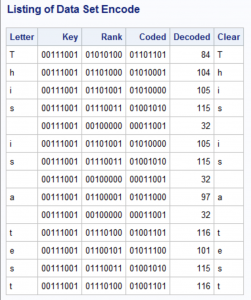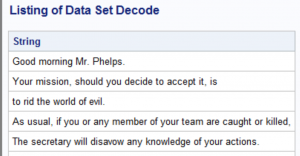There are many reasons why you might want to encrypt data. I use a SAS program to encrypt a list of logon names and passwords. Before we get started describing how to encrypt data, let's discuss some basic concepts concerning encrypting and decrypting data.

All computer data is stored as a series of 1s and 0s. For example, an uppercase A in ASCII is 01000001. Many encrypting schemes use a key to transform plaintext into ciphertext. As an example, suppose your key is B (01000010).

I'm sure you remember the Boolean operators AND, NOT, and OR. If you perform an AND operator on A and B, the result is a 1 if both values are true (1) and false (0) otherwise. So, A AND B is 01000000. The OR operator results in a value of true if either A or B is true, or both A and B are true. Therefore, A OR B is 01000011. The operator that you might not be as familiar with is the exclusive OR (XOR) operator. This is similar to an OR operator except that if both A and B are true, the result is false. A XOR B is equal to 00000011. Why is this useful? An interesting property of the XOR operator is if you take the result and use the XOR operator again on the previous result, you get back to the original value.

The table below shows how the XOR operator works:

 A 0 1 0 0 0 0 0 1 B 0 1 0 0 0 0 1 0 A XOR B 0 0 0 0 0 0 1 1 (A XOR B) XOR B 0 1 0 0 0 0 0 1

If A is the cleartext message and B is the key, A XOR B is the ciphertext. If you perform an exclusive OR with the key (B) and the ciphertext (as was done in the last line), you get back the cleartext value (A).

Of course, you don't use single letter keys to encode messages. Key lengths of 8, 16, or even up to 512 are common. The problem with any key is that if you apply it to a long message, there is a pattern in the ciphertext that allows a code breaker to figure out how long the key is, and even what it is. There are several computer programs that can decode many of the popular encrypting methods. My favorite is:

https://www.geocachingtoolbox.com/

Here is a list of ciphers that can be broken with this program:

 ADFGX/ADFGVX cipher Four-square cipher Substitution cipher Affine cipher Gronsfeld cipher Trifid cipher Atbash cipher Kamasutra cipher Vanity code Bacon cipher Kenny code Vigenère cipher Bifid cipher One-time pad Vigenère cipher decoder Burrows-Wheeler transform Playfair cipher Caeser cipher (ROT13) Rail Fence cipher

Let's write a short SAS program that uses a key to encode a text string.

```data Encode;   retain Key 12345; ❶   length Letter \$ 1; ❷   String = 'This is a test';   do i = 1 to lengthn(String);   Letter = substr(String,i,1); ❸   Rank = rank(Letter); ❹   Coded = bxor(Rank,Key); ❺   Decoded = bxor(Coded,Key); ❻   Clear = byte(Decoded); ❼   output;   end;   drop i;   run;       title "Listing of Data Set Encode";   proc print dta=Encode noobs;   var Letter Key Rank Coded Decoded Clear;   format Key Rank Coded binary8.;   run;```

❶ A RETAIN statement is used to assign the number 12345 to a numeric variable called Key.  (You could have used an assignment statement, but using a RETAIN statement is more efficient and elegant.

❷ The variable Letter will hold each letter of the message and is set to a length of one.

❸ The SUBSTR function will extract each letter from String.

❹ Because Boolean operators only operate on true/false values, you use the RANK function to convert each letter to its ASCII value (stored internally as a series of 0s and 1s).

❺ You now use the BXOR (binary exclusive OR) function to encode each letter of your message.

❻ To demonstrate that the program is working, you use the BXOR function again to demonstrate that this process will return the original String.

❼ The BYTE function takes an ASCII value and returns the appropriate character.

Here is the listing of data set Encode:Because this encryption method uses a single (and short) key, it would be fairly easy to break. What if you encode every letter of the original message with a different key? You can accomplish this by using the SAS random function RAND and using a seed value so that the same series of random numbers gets generated every time you run the program. You can even use one of a dozen different random distributions, to make it harder for someone to decode your file. Here is an example:

First, here is a copy of my text file that contains my secret message (stored in the location c:\Books\Blogs\Cipher\Clear_Text.txt).

Good morning Mr. Phelps.

Your mission, should you decide to accept it,

is to rid the world of evil.

As usual, if you or any member of your team are caught or killed,

the Secretary will disavow any knowledge of your actions.

The following program encrypts this file and creates a temporary data set (in a real situation, you would make this a permanent data set):

```data Coded;   call streaminit(13579); ❶   array l \$ 1 _temporary_; ❷   array num _temporary_; ❸   array xor; ❹   infile "c:\Books\Blogs\Cipher\Clear_Text.txt" pad;   input string \$150.; ❺   do i = 1 to dim(l);   l[i] = substr(string,i,1); ❼   num[i] = rank(l[i]); ❽   xor[i] = bxor(num[i],int(100*rand('Uniform'))); ❾   end;   keep xor1-xor150;   run;```

❶ You need to set a seed value using CALL STREAMINIT so that when you run the decoding program, you will generate the same series of random numbers.

❷ This temporary array will hold up to 150 characters.

❸ The Num array holds the numerical ASCII value for each of the characters in the line.

❹ The XOR array holds the values of the exclusive OR between each numerical ASCII value and the Key.

❺ Read in a string of up to 150 characters.

❻ The DO LOOP picks up each character in a line, starting from 1 and ending at the length of each line.

❼ The RANK function outputs the ASCII value of each character.

❽ The BXOR (binary exclusive OR) function performs the exclusive OR between each ASCII value and the Key.

To decode this message, use the following program:

```data Decode;   call streaminit(13579); ❶   array l \$ 1 _temporary_;   array num _temporary_;   array xor;   length String \$ 150;   set Coded;   do i = 1 to dim(l);   num[i] = bxor(xor[i],int(100*rand('Uniform'))); ❷   l[i] = byte(num[i]); ❸   substr(String,i,1) = l[i]; ❹   end;   keep String;   run;```

❶ Notice that the value of the CALL STREAMINIT routine uses the same seed as the previous program.

❷ The BXOR function between each coded value and the Key, will produce the cleartext.

❸ The BYTE function will convert the ASCII values back to letters, numbers, and other characters.

❹ Finally, the SUBSTR function used on the left-hand side of the equal sign will place each of the characters into the appropriate location in the String variable. (See my previous blog that discusses the use of the SUBSTR function used on the left-hand side of the equal sign.)

Here is the output:It would be straightforward to convert these two programs into macros so that you could encrypt and decrypt any file.

Of course, I would be remiss if I didn't mention that you can encrypt a SAS data set using two data set options ENCRYPT=and ENCRYPTKEY="password". But what would be the fun of that?

Here is an example:

```*Note: AES stands for Advanced Encryption Standard   If you use quotation marks on the ENCRYPTKEY= option, you have   more flexibility in choosing a password (maximum length=64);       data Secret(encrypt=aes encryptkey="mypassword");   input String \$80.;   datalines;   This is a secret message.   See if you can decode it.   This message will not self-destruct!   ;```

You can decode the encrypted data set by including the DATA set option ENCRYPTKEY="password" in any procedure, such as the PROC PRINT shown below:

```title "Listing of Data Set Secret";   proc print data=Secret(encryptkey="mypassword") noobs;   run;```

Encrypting Data Using SAS was published on SAS Users.